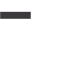# Analytical Questions from the Knowledge Area of Procurement Management

Will you be surprised if PMP exams include questions about calculations from the Procurement Management knowledge area? Don’t worry; they all follow simple logic. Here are some sample questions with answers to help you understand.

Q1: A cost-plus-percentage-cost (CPPC) contract with an agreed-upon profit of 10% of the costs has an estimated cost of \$120,000. The project’s actual cost is \$130,000. What is the total amount repaid to the seller?

Q2: The estimated cost of a cost-plus-incentive-fee (CPIF) contract is \$150,000, with a predetermined fee of \$15,000 and an 80/20 share ratio. The project’s actual cost is \$130,000. What is the seller’s profit margin?

Q3: The target cost of a fixed-price-plus-incentive-fee (FPI) contract is \$130,000, the target profit is \$15,000, the target price is \$145,000, the ceiling price is \$160,000, and the share ratio is 80/20. The project actually cost \$150,000 to complete. What is the seller’s profit margin?

Q4: The target cost of a fixed-price-plus-incentive-fee (FPI) contract is \$150,000, the target profit is \$30,000, the target price is \$180,000, the ceiling price is \$200,000, and the share ratio is 60/40. The project actually cost \$210,000 to complete. Determine the final fee and final price.

Q5: A fixed-price-plus-incentive-fee (FPI) contract has a \$9,000,000 target cost, a \$850,000 target profit, a \$12,500,000 ceiling price, and a 70/30 share ratio. The project actually cost \$8,000,000. Determine the final fee and final price.

Q6: The estimated cost of a cost-plus-incentive-fee (CPIF) contract is \$210,000, with a fee of \$25,000 and an 80/20 share ratio. The project actually cost \$200,000 to complete. Determine the final fee and final price.

Q1: \$120,000 is the estimated cost.

\$130,000 in actual costs

Profit agreed upon=10%

Reimbursement amount = actual cost + % profit on actual cost = \$130,000+

(10% of \$130,000) equals \$143,000

Q2: \$150,000 is the estimated cost.

A fee fixed at \$15,000

80/20 share ratio ( 80 is for the Buyer and 20 is for the seller)

\$130,000 in actual costs

Saving = estimated cost minus actual cost = \$20,000

The seller’s profit is Predetermined fee + (Seller’s share ratio * Savings) = \$15,000 + (20% * \$20,000) = \$19,000

Q3: \$130,000 budgeted cost

Fee Estimated at \$15,000

\$145,000 is the target price.

\$160,000 is the maximum price.

80/20 share ratio ( 80 is for the Buyer and 20 is for the seller)

Actual cost is \$150,000

In this case, the actual cost is less than the ceiling price but greater than the target cost.

Final Fee = ((Target Cost-Actual Cost) * Seller Ratio) + Target fee = ((\$130,000-\$150,000)*20 percent +\$15,000=(-\$20,000*20 percent)+\$15,000=-\$4,000+\$15,000=\$11,000 Final Price = Actual Cost + Final Fee = \$150,000 + \$11,000 = \$161,000. However, this is more than the ceiling price of \$160,000. As a result, the seller receives a final price of \$160,000.

So the seller’s profit is \$160,000-\$150,000= \$10,000.

Q4: \$150,000 budgeted cost

Fee Estimated at \$30,000

\$180,000 is the target price.

The maximum price is \$200,000

60/40 share ratio (60 is for the Buyer and 40 is for the seller)

Actual cost is \$210,000.

In this case, the actual cost is greater than the target price and also greater than the ceiling price. As a result, the seller is in trouble. Let’s see what he gets.

Final Fee=((Target Cost-Actual Cost)*Seller Ratio) + Target fee=((150,000-\$210,000)*40 percent +\$30,000=(-\$60,000*40 percent)+\$30,000=-\$24,000+\$30,000=\$6,000

Final Price = Actual Cost + Final Fee = \$210,000 + 6,000 = \$216,000. However, this exceeds the ceiling price. As a result, the final cost is \$200,000 J.

Q5: The estimated cost is \$9,000,000.

Target Fee: \$850,000

Target price is \$9,850,000.

The maximum price is \$12,500,000

70/30 share ratio

(70 for the buyer, 30 for the seller)

Actual cost is \$8,000,000.

In this case, the actual cost is less than the target price and less than the ceiling price. Let’s see how much the seller receives.

Final Fee = ((Target Cost-Actual Cost)*Seller Ratio) + Target Fee = ((\$9,000,000- \$8,000,000)*30%)

+\$850,000=(\$1,000,000*30% of \$1,000,000)

+\$850,000= \$300,000 +\$850,000= \$1,150,000

Final Price = Actual Cost + Final Fee = \$8,000,000 + \$1,150,000 = \$9,150,000

Q6: Approximate cost = \$210,000

Fee fixed at \$25,000

80/20 share ratio (80 is for the Buyer and 20 is for the seller)

The actual cost is \$200,000

Saving = Estimated Cost minus Actual Cost = \$10,000

Final Fee= (Saving * Seller Ratio) + Predetermined Fee=(\$10,000*20%)+\$25,000=\$2,000+\$25,000= \$27,000

Final Cost=Actual Cost + Final Fee=\$200,000+\$27,000 =\$227,000

Point of Total Assumption (PTA): This applies only to fixed-price incentive fee contracts and refers to the amount above which the seller bears the entire cost overrun loss. Mismanagement is to blame for this. Sellers will occasionally compare their actual costs to the PTA to ensure they are still profiting from the project.